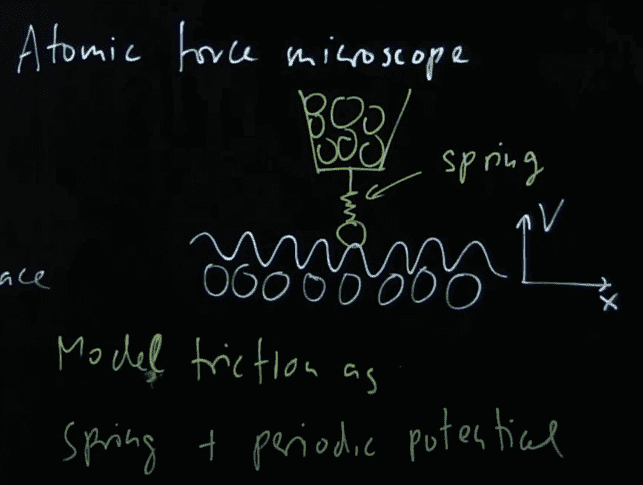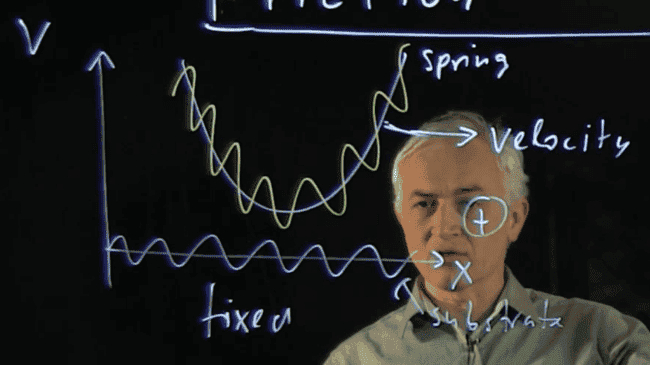# Understanding the Tomlinson Model of Sliding Friction

Leo Liu
I have just watched a video lecture about the Tomlinson model (aka Prandtl–Tomlinson Model) which explains the friction mechanism at a nanoscale by analogy with a tip dragged by a spring. The video has prompted me to ask several questions.The spring is dragged over a corrugated substrate, as shown in the picture above. Because of the corrugation, the potential energy curve of the substrate is periodic.
However, I am not quite sure why there is some potential energy between the nanotip and the substrate-- my guess is that the potential energy is stored within the intermolecular forces between the tip and the molecules in the substrate. Could someone provide an explanation?Then he mentions that the curve of the potential energy of the spring is quadratic. Because the length of the spring is constantly changing, I wonder why the EP curve of the spring does not show any periodicity.Last, why is the corrugation of the potential energy proportional to the normal force?

I would also like to invite you to share your knowledge and insight into this model or the mechanism of friction on the atomic scale. Thank you.

Last edited:
•etotheipi

Hey Leo, it's quite an interesting topic! As far as I can tell, we take the extension of the spring to be approximately the horizontal displacement of the point of attachment (moving at ##v##) from the position of the particle, resulting in an elastic potential energy of ##\frac{1}{2}k(x-vt)^2##. We model the total potential energy as $$V = V_{\text{interaction}} + V_{\text{elastic}} = V_0\cos{\left(\frac{2\pi}{a}x \right)} + \frac{1}{2}k(x-vt)^2$$if ##x## is the position of the particle, ##a## the interatomic spacing of the substrate, and ##v## the speed of the tip. That is the squiggly potential he has drawn. Also, you must certainly be correct that the first term is due to electric interactions with the substrate. If we also add in a damping force ##-\eta \vec{v}## then the differential equation describing the motion is$$m\ddot{x} = k(vt-x) - N\sin{\left(\frac{2\pi}{a} x \right)} -\eta \dot{x}$$with ##N = -\frac{2\pi V_0}{a}##. From this you can derive a few special cases for the friction, as is nicely explained here, although they use ##F## for the external force on the particle from the spring, which I have called ##k(vt - x)## so that it is more general than just the case of uniform relative velocity.

However I do not know why the potential energy is proportional to the normal force. I too would be interested in a justification for this!

Last edited by a moderator:
•Leo Liu
Leo Liu
Hey Leo, it's quite an interesting topic! As far as I can tell...
Hey James. I am not sure what you meant by "damping force". For a simple harmonic oscillator in real life, the dumping force is basically the friction force. However, since we are talking about the essence of kinetic friction here, the macroscopic friction force should not exist at a nanoscale. I would be grateful if you can inform me what the dumping force is in the equation you wrote.

Redrafting after thinking about it a bit more...

The damping term is there to account for dissipation of heat. A bit more digging tells me that this is actually an example of a Langevin equation and is really within the realm of statistical mechanics.

I cannot add much more, since I too am struggling to make out what is happening. I am going through this reference: http://www.iop.kiev.ua/~obraun/book_md/book_md_4.pdf

Last edited by a moderator:
Leo Liu
Redrafting after thinking about it a bit more...

The damping term is there to account for dissipation of heat. A bit more digging tells me that this is actually an example of a Langevin equation and is really within the realm of statistical mechanics.

I cannot add much more, since I too am struggling to make out what is happening. I am going through this reference: http://www.iop.kiev.ua/~obraun/book_md/book_md_4.pdf
Everything on a microscope level behaves differently than the macroscopic objects. Perhaps it is insanity to apply our intuition based on large objects to the behaviour of tiny particles.

Leo Liu
However I do not know why the potential energy is proportional to the normal force. I too would be interested in a justification for this!
I think I have found an answer to this question in Kleppner's mechanics book.
An Introduction to Mechanics said:
"In many cases the maximum value of the friction between two surfaces is found to be essentially independent of the area of contact, which may seem strange. The reason is that the actual area of contact on an atomic scale is a minute fraction of the total surface area. This fraction is proportional to the pressure, that is, the force per unit area. If the areais doubled while the normal force is held constant, then the pressure is halved. Thus there is twice as much contact area, but only half as much microscopic force on each. The resultant total friction force, the product of contact area and microscopic force, is unchanged. This is an oversimplified explanation and non-rigid bodies like automobile tires are more complicated. A wide tire is generally better than a narrow one for good acceleration and braking."

•etotheipi